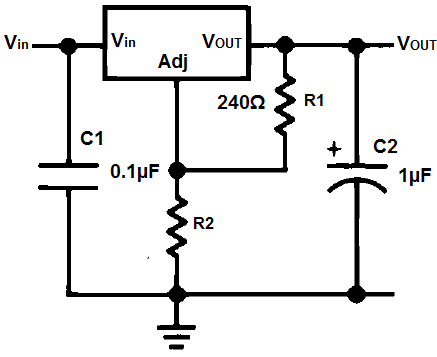﻿ LM317 Resistor and Voltage Calculator ﻿# LM317 Resistor and Voltage CalculatorLM317 FormulaThe LM317 is an adjustable voltage regulator which can output a range of voltages (1.5 to 37V) based on the resistors R1 and R2. Normally the value of R1 is 240Ω, the recommended value by the manufacturer. However, the value of R1 may be any value from 100Ω to 1000Ω. The resistor R2 then is the chief resistor which allows for the swings in different voltage outputs. The formula is below.

### LM317 Voltage Calculator

Enter Resistor, R1:

Enter Resistor, R2:

VOUT: 0.00V

The LM317 Voltage Calculator calculates the output voltage, VOUT based on the values of resistors, R1 and R2, according to the above formula.

A user enters in R1 and R2 and the result will automatically be calculated and shown. The voltage result which is displayed above is in unit volts (V).

You can see by default that resistor R1 is 240Ω, again, as the value which the manufacturuer specifies as the recommended default value. However, you switch out the value if necessary, so this is not a fixed value. It's adjustable.

Example calculation: An example calculation using this calculator is having R1=240Ω and having R2=720Ω. This will produce an output voltage of exactly 5V, being that VOUT= 1.25V * (1+ R2/R1)= 1.25V * (1 + 720Ω/240Ω)= 5V.

### LM317 Resistor Calculator

Enter Resistor, R1:

Enter Voltage, VOUT:

R2: 0.00Ω

The LM317 Resistor Calculator calculates the value of resistor, R2, based on the values of resistor, R1, and the output voltage, VOUT.

A user enters in R1 and VOUT and the result will automatically be calculated and shown. The resistance result which is displayed above is in unit ohms (Ω).

Again, you can see that the value that resistor R1 is by default is 240Ω, as the value which the manufacturer specifies as the recommended default value. However, you can switch out the value if necessary, so this is not a fixed value. It's adjustable.

Example calculation: An example calculation using this calculator is having R1=240Ω and VOUT= 5V. This will produce an output voltage of exactly 5V, being that VOUT= R1 * (VOUT - 1.25V)/1.25V)= 240Ω * ((5V-1.25V)/1.25V)= 720Ω.

Related Resources

﻿